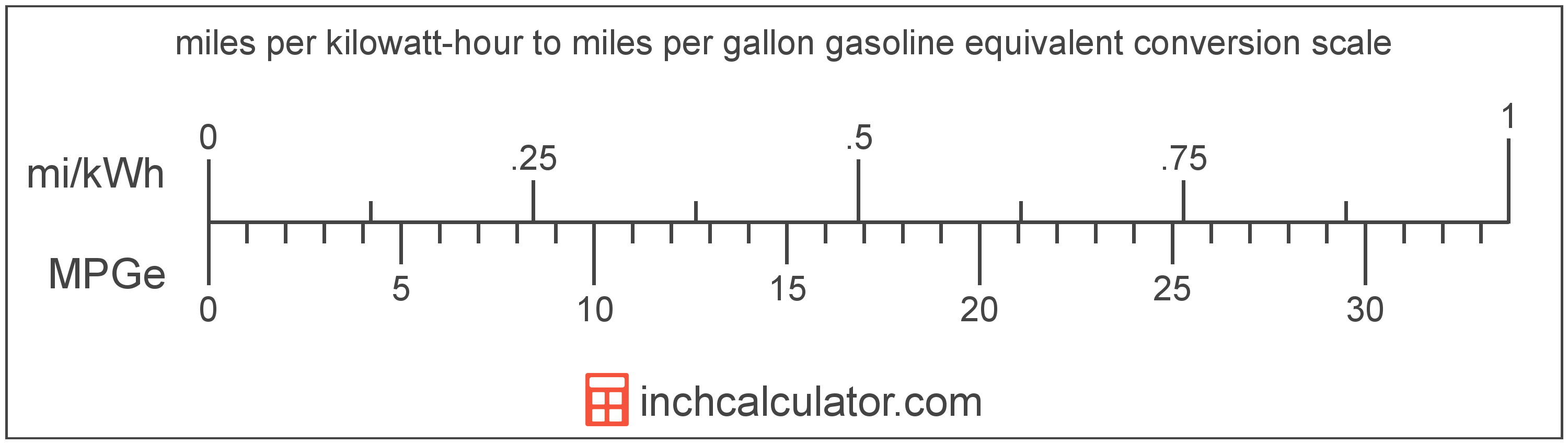# Miles Per Kilowatt-hour to Miles Per Gallon Gasoline Equivalent Conversion (Mi/kWh to MPGe)

Enter the electric car efficiency in miles per kilowatt-hour below to get the value converted to miles per gallon gasoline equivalent.

Results in Miles Per Gallon Gasoline Equivalent:1 mi/kWh = 33.705 MPGe
Do you want to convert MPGe to mi/kWh?

## How to Convert Miles Per Kilowatt-hour to Miles Per Gallon Gasoline EquivalentConvert miles per kilowatt-hour to miles per gallon gasoline equivalent with this simple formula:

MPGe = mi/kWh × 33.705

Insert the mi/kWh efficiency measurement in the formula and then solve to find the result.

For example, let's convert 50 mi/kWh to MPGe:

50 mi/kWh = ( 50 × 33.705 ) = 1,685.25 MPGe

Miles per kilowatt-hour and miles per gallon gasoline equivalent are both units used to measure electric car efficiency. Keep reading to learn more about each unit of measure.

## Miles Per Kilowatt-hour

Miles per kilowatt-hour is a measure of electric vehicle efficiency equal to the distance traveled in miles consuming one kilowatt-hour of energy.

The mile per kilowatt-hour is a US customary unit of electric car efficiency. Miles per kilowatt-hour can be abbreviated as mi/kWh; for example, 1 mile per kilowatt-hour can be written as 1 mi/kWh.

In formal expressions, the slash, or solidus (/), is used to separate units used to indicate division in an expression.

## Miles Per Gallon Gasoline Equivalent

Miles per gallon gasoline equivalent is a way of measuring the energy needed equivalent to a gallon of gasoline to travel a distance in miles.

Because electric vehicles do not consume gasoline, the EPA set an equivalent amount of energy in kilowatt-hours that is equal to one gallon of gasoline. According to the EPA, one gallon of gasoline is equal to 33.705 kilowatt-hours.

The mile per gallon gasoline equivalent is a US customary unit of electric car efficiency. Miles per gallon gasoline equivalent can be abbreviated as MPGe; for example, 1 mile per gallon gasoline equivalent can be written as 1 MPGe.

## Mile Per Kilowatt-hour to Mile Per Gallon Gasoline Equivalent Conversion Table

Mile per kilowatt-hour measurements converted to miles per gallon gasoline equivalent
Miles Per Kilowatt-hour Miles Per Gallon Gasoline Equivalent
1 mi/kWh 33.71 MPGe
2 mi/kWh 67.41 MPGe
3 mi/kWh 101.12 MPGe
4 mi/kWh 134.82 MPGe
5 mi/kWh 168.53 MPGe
6 mi/kWh 202.23 MPGe
7 mi/kWh 235.94 MPGe
8 mi/kWh 269.64 MPGe
9 mi/kWh 303.35 MPGe
10 mi/kWh 337.05 MPGe
11 mi/kWh 370.76 MPGe
12 mi/kWh 404.46 MPGe
13 mi/kWh 438.17 MPGe
14 mi/kWh 471.87 MPGe
15 mi/kWh 505.58 MPGe
16 mi/kWh 539.28 MPGe
17 mi/kWh 572.99 MPGe
18 mi/kWh 606.69 MPGe
19 mi/kWh 640.4 MPGe
20 mi/kWh 674.1 MPGe
21 mi/kWh 707.81 MPGe
22 mi/kWh 741.51 MPGe
23 mi/kWh 775.22 MPGe
24 mi/kWh 808.92 MPGe
25 mi/kWh 842.63 MPGe
26 mi/kWh 876.33 MPGe
27 mi/kWh 910.04 MPGe
28 mi/kWh 943.74 MPGe
29 mi/kWh 977.45 MPGe
30 mi/kWh 1,011 MPGe
31 mi/kWh 1,045 MPGe
32 mi/kWh 1,079 MPGe
33 mi/kWh 1,112 MPGe
34 mi/kWh 1,146 MPGe
35 mi/kWh 1,180 MPGe
36 mi/kWh 1,213 MPGe
37 mi/kWh 1,247 MPGe
38 mi/kWh 1,281 MPGe
39 mi/kWh 1,314 MPGe
40 mi/kWh 1,348 MPGe

## References

1. United States Environmental Protection Agency, Technology, https://www3.epa.gov/otaq/gvg/learn-more-technology.htm